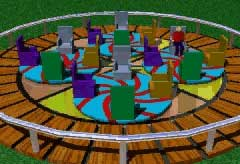Ch 5. Rigid Body General Motion Multimedia Engineering Dynamics Fixed Axis Rotation Plane Motion Velocities Zero Velocity Point Plane Motion Accelerations Multiple Gears Rot. Coord. Velocities Rot. Coord. Acceleration
 Chapter - Particle - 1. General Motion 2. Force & Accel. 3. Energy 4. Momentum - Rigid Body - 5. General Motion 6. Force & Accel. 7. Energy 8. Momentum 9. 3-D Motion 10. Vibrations Appendix Basic Math Units Basic Equations Sections Search eBooks Dynamics Fluids Math Mechanics Statics Thermodynamics Author(s): Kurt Gramoll ©Kurt GramollDYNAMICS - CASE STUDY IntroductionProblem Graphic Fun Time, Inc., has just designed a new amusement-park ride based on the spinning disk concept. In order to operate the new ride, the velocity at different times is needed. What is known: The main disk rotates at Ω = 1 rad/s (θ = Ωt). The rider disk rotates at ω = 2 rad/s (β = ωt). Both angular velocities are constant. Question What is the velocity of the rider, shown in the dynamic diagram at left, at 2.4 seconds? Approach Use the relative velocity equation for rotating coordinates, vB = vA + Ω × rB/A + vB/A

Practice Homework and Test problems now available in the 'Eng Dynamics' mobile app
Includes over 400 problems with complete detailed solutions.
Available now at the Google Play Store and Apple App Store.